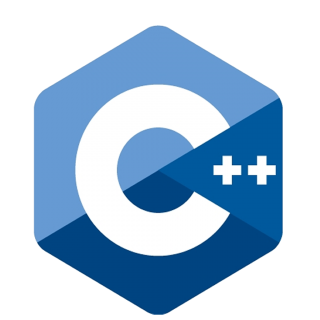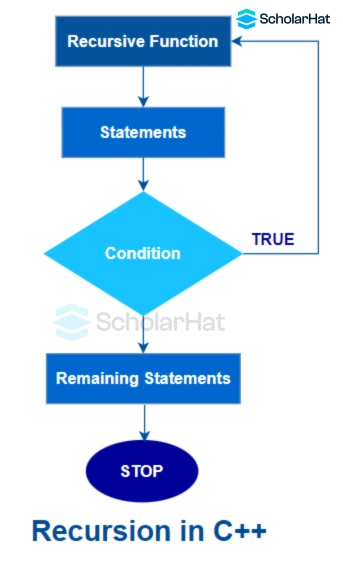# What is Recursion in C++ | Types of Recursion in C++ ( With Examples )

04 Dec 2023
Intermediate
191 ViewsLearn via Video Course & by Doing Hands-on Labs

## Recursion in C++: An Overview

In the previous tutorials, we went through various aspects of functions in C++like `function parameters`, call by value and call by reference, etc. Hence, we have become familiar with `functions` and its uses in programming. So, in this C++ tutorial, we are now moving towards `recursion`, a little advanced but very important technique in C++ programming. You can even check out our C++ Certification program for more understanding.

## What is Recursion in C++?

Recursion is the process in which a `function` calls itself again and again. It entails decomposing a challenging issue into more manageable issues and then solving each one again. There must be a `terminating condition` to stop such `recursive calls`.#### Syntax

``````recursionfunction()
{
recursionfunction(); //calling self function
}
``````

#### Example

``` ```#include <iostream>
using namespace std;
int fibonacci(int);

int main() {
int n, f;
n=12;
f = fibonacci(n);
cout << f;
return 0;
}

int fibonacci(int n) {
if (n == 0) {
return 0;
} else if (n == 1) {
return 1;
} else {
return fibonacci(n - 1) + fibonacci(n - 2);
}
}
``````
• This C++ program uses `recursion` to determine the `nth` Fibonacci number.
• It accepts the input `n` and returns 0 or 1 depending on whether `n` is 0 or 1.
• Otherwise, it adds the two Fibonacci numbers before calculating the Fibonacci number recursively.

#### Output

``````if n = 12 then output will be:
144``````

• The code can be made shorter with the help of `recursion`.
• It offers a clear and straightforward method for writing the code.
• It reduces the need to call the function repeatedly.
• In issues like tree traversals as well as the`Tower of Hanoi`, `recursion` is recommended.

• Slower than non-recursive functions.
• The code is difficult to decipher or comprehend.
• Debugging can be difficult as compared to iterative programs.
• Its space requirements are more compared to iterative programs.
• Due to `function calls`, it requires more time than usual.
##### Summary

`Recursion`is a very useful approach when it comes to programming. It has wide applications ranging from calculating the factorial of a number to sorting and traversal algorithms. If you want to learn such an important concept in more depth, just consider our C++Certification Program. It will prove a practical booster in your journey of programming.

Share Article
Similar Articles